SSC CGL Previous Year Questions: Time, Speed & Distance (Boat and Streams) - 2

# SSC CGL Previous Year Questions: Time, Speed & Distance (Boat and Streams) - 2 - SSC CGL

Test Description

## 30 Questions MCQ Test SSC CGL (Tier - 1) - Previous Year Papers (Topic Wise) - SSC CGL Previous Year Questions: Time, Speed & Distance (Boat and Streams) - 2

SSC CGL Previous Year Questions: Time, Speed & Distance (Boat and Streams) - 2 for SSC CGL 2023 is part of SSC CGL (Tier - 1) - Previous Year Papers (Topic Wise) preparation. The SSC CGL Previous Year Questions: Time, Speed & Distance (Boat and Streams) - 2 questions and answers have been prepared according to the SSC CGL exam syllabus.The SSC CGL Previous Year Questions: Time, Speed & Distance (Boat and Streams) - 2 MCQs are made for SSC CGL 2023 Exam. Find important definitions, questions, notes, meanings, examples, exercises, MCQs and online tests for SSC CGL Previous Year Questions: Time, Speed & Distance (Boat and Streams) - 2 below.
Solutions of SSC CGL Previous Year Questions: Time, Speed & Distance (Boat and Streams) - 2 questions in English are available as part of our SSC CGL (Tier - 1) - Previous Year Papers (Topic Wise) for SSC CGL & SSC CGL Previous Year Questions: Time, Speed & Distance (Boat and Streams) - 2 solutions in Hindi for SSC CGL (Tier - 1) - Previous Year Papers (Topic Wise) course. Download more important topics, notes, lectures and mock test series for SSC CGL Exam by signing up for free. Attempt SSC CGL Previous Year Questions: Time, Speed & Distance (Boat and Streams) - 2 | 30 questions in 30 minutes | Mock test for SSC CGL preparation | Free important questions MCQ to study SSC CGL (Tier - 1) - Previous Year Papers (Topic Wise) for SSC CGL Exam | Download free PDF with solutions
 1 Crore+ students have signed up on EduRev. Have you?
SSC CGL Previous Year Questions: Time, Speed & Distance (Boat and Streams) - 2 - Question 1

### A man goes to a place on bicycle at speed of 16 km/hr and comes back at lower speed. If the average speed is 6.4 km/hr in total, then the return speed (in km/hr) is    (SSC CGL 1st Sit. 2016)

Detailed Solution for SSC CGL Previous Year Questions: Time, Speed & Distance (Boat and Streams) - 2 - Question 1

Average speed when speed x and y are given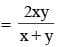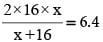32 x = 6.4(x + 16)
x = 0.2(x + 16)
0.8x = 3.2
x = 4

SSC CGL Previous Year Questions: Time, Speed & Distance (Boat and Streams) - 2 - Question 2

### A train 180 m long is running at a speed of 90 km/hr. How long will it take to pass a post?    (SSC CGL 1st Sit. 2015)

Detailed Solution for SSC CGL Previous Year Questions: Time, Speed & Distance (Boat and Streams) - 2 - Question 2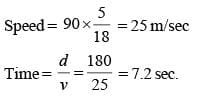SSC CGL Previous Year Questions: Time, Speed & Distance (Boat and Streams) - 2 - Question 3

### A train runs at an average speed of 75 km/hr. If the distance to be covered is 1050 km. How long will the train take to cover it?    (SSC CGL 1st Sit. 2015)

Detailed Solution for SSC CGL Previous Year Questions: Time, Speed & Distance (Boat and Streams) - 2 - Question 3

Average speed = 75km/h
Distance = 1050 km
Time taken to cover the distance = 1050/75 =14 hrs.

SSC CGL Previous Year Questions: Time, Speed & Distance (Boat and Streams) - 2 - Question 4

Walking at the rate of 4 kmph a man covers certain distance in 2hrs 45 min. Running at a speed of 16.5 kmph the man will cover the same distance in how many minutes?    (SSC CGL 1st Sit. 2015)

Detailed Solution for SSC CGL Previous Year Questions: Time, Speed & Distance (Boat and Streams) - 2 - Question 4

When distance is contant, then speed is inversely proportional
S1 : S2 = T2 : T1
4 : 16.5  = T2 : 165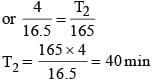SSC CGL Previous Year Questions: Time, Speed & Distance (Boat and Streams) - 2 - Question 5

A farmer travelled a distance of 61 km in 9 hrs. He travelled partly on foot at the rate of 4 km/hr and partly on bicycle at the rate of 9 km/hr. The distance travelled on foot is     (SSC CGL 1st Sit. 2015)

Detailed Solution for SSC CGL Previous Year Questions: Time, Speed & Distance (Boat and Streams) - 2 - Question 5

Let the distance travelled as foot be x km.
Then, distance travelled by bicycle  = (61 –x) km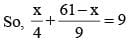9x + 4 (61 –x) = 9 × 36
9x – 4x = 324 – 244
5x = 80
x = 16 km

SSC CGL Previous Year Questions: Time, Speed & Distance (Boat and Streams) - 2 - Question 6

A ship after sailing 12km towards south from a particular place covered 5 km more towards east. Then the straightway distance of the ship from that place is    (SSC CGL 1st Sit. 2015)

Detailed Solution for SSC CGL Previous Year Questions: Time, Speed & Distance (Boat and Streams) - 2 - Question 6

Given, AB = 12 km, BC = 5 km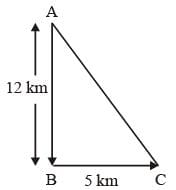Straight way distance of ship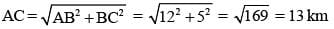SSC CGL Previous Year Questions: Time, Speed & Distance (Boat and Streams) - 2 - Question 7

The speed of a car is 54 km/hr. What is its speed in m/sec?   (SSC CHSL 2015)

Detailed Solution for SSC CGL Previous Year Questions: Time, Speed & Distance (Boat and Streams) - 2 - Question 7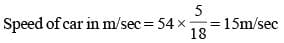SSC CGL Previous Year Questions: Time, Speed & Distance (Boat and Streams) - 2 - Question 8

A train 150 m long passes a pole in 30 seconds and another train of the same length travelling in opposite direction in 10 seconds. The speed of the second train is:    (SSC CHSL 2015)

Detailed Solution for SSC CGL Previous Year Questions: Time, Speed & Distance (Boat and Streams) - 2 - Question 8

Speed of first train = 150/30 = 5m/sec
Let the speed of second train be x  m/sec
Relative speed = (5 + x) m /sec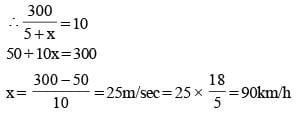SSC CGL Previous Year Questions: Time, Speed & Distance (Boat and Streams) - 2 - Question 9

How many seconds will a train 120 metres long running the rate of 36km/ hr take to cross a bridge of 360 metres in length?    (SSC Sub. Ins. 2015)

Detailed Solution for SSC CGL Previous Year Questions: Time, Speed & Distance (Boat and Streams) - 2 - Question 9

Total distance = Length of train + Length of bridge = 120 + 360 = 480 m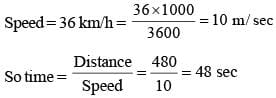SSC CGL Previous Year Questions: Time, Speed & Distance (Boat and Streams) - 2 - Question 10

A bus moving at 40km per hour covers a distance in 6 hours 15 minutes. If it travels the same distance at 50 km per hour how long will it take to cover the distance?    (SSC Sub. Ins. 2015)

Detailed Solution for SSC CGL Previous Year Questions: Time, Speed & Distance (Boat and Streams) - 2 - Question 10

Distance covered = Speed × Time =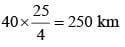New speed = 50 km/hour
∴ Time taken to cover same distance = 250/50 = 5h

SSC CGL Previous Year Questions: Time, Speed & Distance (Boat and Streams) - 2 - Question 11

If a train runs at 70 km/hour, it reaches its destination late by 12 minutes. But if it runs at 80 km/hour, it is late by 3 minutes.
The correct time to cover the journey is    (SSC CGL 2014)

Detailed Solution for SSC CGL Previous Year Questions: Time, Speed & Distance (Boat and Streams) - 2 - Question 11

Let correct time to cover journey be t hours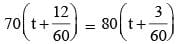70t + 14 = 80t+  4
10t = 10
t = 1 hour

SSC CGL Previous Year Questions: Time, Speed & Distance (Boat and Streams) - 2 - Question 12

It takes 8 hours for a 600 km journey, if 120 km is done by train and the rest by car. It takes 20 minutes more if 200 km is down by train and the rest by car. The ratio of the speed of the train to that of the car is    (SSC CGL 2014)

Detailed Solution for SSC CGL Previous Year Questions: Time, Speed & Distance (Boat and Streams) - 2 - Question 12

Let T be the speed of train and C be the speed of car.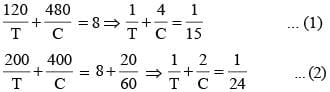Subtracting (2) from (1)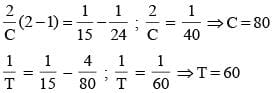Required ratio = 60 : 80 = 3 : 4

SSC CGL Previous Year Questions: Time, Speed & Distance (Boat and Streams) - 2 - Question 13

A horse take 2½ seconds to complete a round around a circular field. If the speed of the horse was 66 m/sec, then the radius of the field is, [Given π = 22/7]    (SSC CHSL 2014)

Detailed Solution for SSC CGL Previous Year Questions: Time, Speed & Distance (Boat and Streams) - 2 - Question 13

Total distance covered by horse in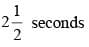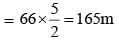Radius of the field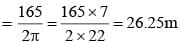SSC CGL Previous Year Questions: Time, Speed & Distance (Boat and Streams) - 2 - Question 14

A is twice as fast as B and B is thrice as fast as C is. The journey covered by C in 1½ hours will be covered by A in    (SSC CHSL 2014)

Detailed Solution for SSC CGL Previous Year Questions: Time, Speed & Distance (Boat and Streams) - 2 - Question 14

Let C’s speed = x km/h
Then, B’s speed = 3x km/h
and A’s speed = 6x km/h
Ratio of speeds of A, B, C = 6x : 3x : x = 6 : 3 : 1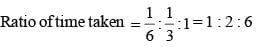It C’s 90 minutes
Hence, 6x = 90
x = 15 minutes
Hence, A should take 15 minutes.

SSC CGL Previous Year Questions: Time, Speed & Distance (Boat and Streams) - 2 - Question 15

A train 50 metre long passes a platform 100 metre long in 10 sec. The speed of the train in km/hr is    (SSC Sub. Ins. 2014)

Detailed Solution for SSC CGL Previous Year Questions: Time, Speed & Distance (Boat and Streams) - 2 - Question 15

Total distance = (100 + 50) m = 150 m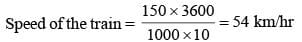SSC CGL Previous Year Questions: Time, Speed & Distance (Boat and Streams) - 2 - Question 16

A student goes to school at the rate of 5/2 km/hr and reaches 6 minutes late. If he travels at the speed of 3 km/hr, he reaches 10 minutes earlier. The distance of the school is    (SSC Sub. Ins. 2014)

Detailed Solution for SSC CGL Previous Year Questions: Time, Speed & Distance (Boat and Streams) - 2 - Question 16

Let original time taken by student be x hours.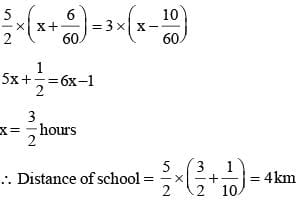SSC CGL Previous Year Questions: Time, Speed & Distance (Boat and Streams) - 2 - Question 17

Raju has to cover a distance of 240 km in 4 hours. If he covers one-third of the journey in 2/7th time, what is his speed at the beginning of the journey?    (SSC Multitasking 2014)

Detailed Solution for SSC CGL Previous Year Questions: Time, Speed & Distance (Boat and Streams) - 2 - Question 17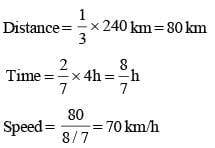SSC CGL Previous Year Questions: Time, Speed & Distance (Boat and Streams) - 2 - Question 18

A railway train 100 metres long is running at the speed of 30 km/hr. In what time does it pass a man standing near a line?    (SSC Multitasking 2014)

Detailed Solution for SSC CGL Previous Year Questions: Time, Speed & Distance (Boat and Streams) - 2 - Question 18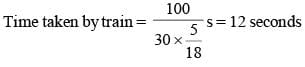SSC CGL Previous Year Questions: Time, Speed & Distance (Boat and Streams) - 2 - Question 19

By walking at 3/4 of his usual speed, a man reaches his office 20 minutes later than usual. His usual time is    (SSC CGL 1st Sit. 2013)

Detailed Solution for SSC CGL Previous Year Questions: Time, Speed & Distance (Boat and Streams) - 2 - Question 19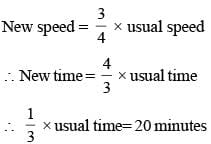⇒ Usual time = 3 × 20 = 60 minutes

SSC CGL Previous Year Questions: Time, Speed & Distance (Boat and Streams) - 2 - Question 20

A man performs 2/15 of the total journey by train, 9/20 by bus and the remaining 10 km on foot. His total journey in km is    (SSC CGL 1st Sit. 2013)

Detailed Solution for SSC CGL Previous Year Questions: Time, Speed & Distance (Boat and Streams) - 2 - Question 20

If the total journey be of x km, then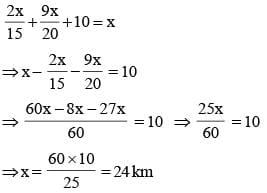SSC CGL Previous Year Questions: Time, Speed & Distance (Boat and Streams) - 2 - Question 21

A train 100 metres long moving at a speed of 50 km/hr. crosses a train 120 metres long coming from opposite direction in 6 sec. The speed of the second train is    (SSC CGL 1st Sit. 2013)

Detailed Solution for SSC CGL Previous Year Questions: Time, Speed & Distance (Boat and Streams) - 2 - Question 21

Let speed of the second train = x km/hr.
Relative speed of trains = (50 + x) km/hr.
Distance travelled by trains = (100 + 120) = 220 metres
Distance = Speed × Time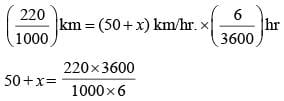50 + x = 132
x = 132 – 50 = 82 km/hr

SSC CGL Previous Year Questions: Time, Speed & Distance (Boat and Streams) - 2 - Question 22

Anil calculated that it will take 45 minutes to cover a distance of 60 km by his car. How long will it take to cover the same distance if the speed of his car is reduced by 15 km/hr?   (SSC CGL 1st Sit. 2013)

Detailed Solution for SSC CGL Previous Year Questions: Time, Speed & Distance (Boat and Streams) - 2 - Question 22

D = S × T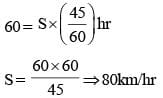Now, new speed = 80 – 15 = 65 km/hr.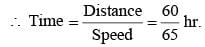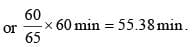Hence, Time to taken by car to travel same distance is 55.38 min.

SSC CGL Previous Year Questions: Time, Speed & Distance (Boat and Streams) - 2 - Question 23

A certain distance is covered at a certain speed. If half of this distance is covered in double the time, the ratio of the two speeds is    (SSC CGL 1st Sit. 2013)

Detailed Solution for SSC CGL Previous Year Questions: Time, Speed & Distance (Boat and Streams) - 2 - Question 23

If the original speed be S1 units and time = t1 units and distance = D, then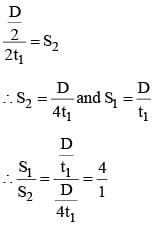SSC CGL Previous Year Questions: Time, Speed & Distance (Boat and Streams) - 2 - Question 24

Two cars are moving with speeds v1, v2 towards a crossing along two roads. If their distances from the crossing be 40 metres and 50 metres at an instant of time then they do not collide if their speeds are such that    (SSC CGL 1st Sit. 2013)

Detailed Solution for SSC CGL Previous Year Questions: Time, Speed & Distance (Boat and Streams) - 2 - Question 24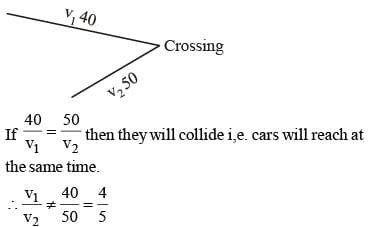SSC CGL Previous Year Questions: Time, Speed & Distance (Boat and Streams) - 2 - Question 25

A man rows down a river 15 km in 3 hrs. with the stream and returns in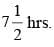The rate at which he rows in still water is

Detailed Solution for SSC CGL Previous Year Questions: Time, Speed & Distance (Boat and Streams) - 2 - Question 25

Speed of person in still water = x kmph and speed of current = y kmph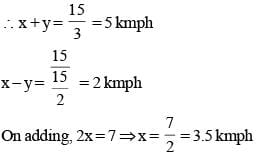SSC CGL Previous Year Questions: Time, Speed & Distance (Boat and Streams) - 2 - Question 26

A man rides at the rate of 18 km/hr, but stops for 6 mins. to change horses at the end of every 7th km. The time that he will take to cover a distance of 90 km is    (SSC CGL 2nd Sit.  2013)

Detailed Solution for SSC CGL Previous Year Questions: Time, Speed & Distance (Boat and Streams) - 2 - Question 26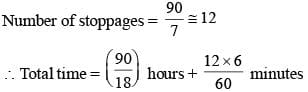= 6 hours 12 minutes

SSC CGL Previous Year Questions: Time, Speed & Distance (Boat and Streams) - 2 - Question 27

A train moving at a rate of 36 km/hr. crosses a standing man in 10 seconds. It will cross a platform 55 metres long, in:    (SSC CGL 1st Sit. 2013)

Detailed Solution for SSC CGL Previous Year Questions: Time, Speed & Distance (Boat and Streams) - 2 - Question 27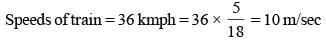Length of train = 10 × 10 = 100 metre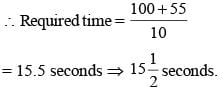SSC CGL Previous Year Questions: Time, Speed & Distance (Boat and Streams) - 2 - Question 28

A man can swim 3 km/hr. in still water. If the velocity of the stream is 2 km/hr. The time taken by him to swim to a place 10 km upstream and back is:    (SSC CGL 1st Sit. 2013)

Detailed Solution for SSC CGL Previous Year Questions: Time, Speed & Distance (Boat and Streams) - 2 - Question 28

Downstream speed = 5 kmph
Upstream speed = 1 kmph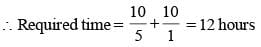SSC CGL Previous Year Questions: Time, Speed & Distance (Boat and Streams) - 2 - Question 29

A train is moving at a speed of 80 km/h and covers a certain distance in 4.5 hours. The speed of the train to cover the same distance in 4 hours is    (SSC CHSL 2013)

Detailed Solution for SSC CGL Previous Year Questions: Time, Speed & Distance (Boat and Streams) - 2 - Question 29

Distance covered in 4.5 h = 80 × 4.5 = 360 km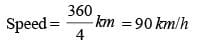SSC CGL Previous Year Questions: Time, Speed & Distance (Boat and Streams) - 2 - Question 30

Water flows at the rate of 10 metres per minute from a cylindrical pipe 5 mm in diameter. How long it take to fill up a conical vessel whose diameter at the base is 30 cm and depth 24 cm?   (SSC Sub. Ins. 2013)

Detailed Solution for SSC CGL Previous Year Questions: Time, Speed & Distance (Boat and Streams) - 2 - Question 30

Volume of water flowing from the pipe in 1 minute
= π x 0.25 x 0.25 x 1000 cu.cm.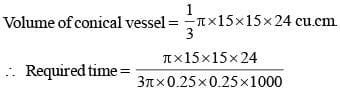= 28 minutes 48 seconds

## SSC CGL (Tier - 1) - Previous Year Papers (Topic Wise)

250 tests
Information about SSC CGL Previous Year Questions: Time, Speed & Distance (Boat and Streams) - 2 Page
In this test you can find the Exam questions for SSC CGL Previous Year Questions: Time, Speed & Distance (Boat and Streams) - 2 solved & explained in the simplest way possible. Besides giving Questions and answers for SSC CGL Previous Year Questions: Time, Speed & Distance (Boat and Streams) - 2, EduRev gives you an ample number of Online tests for practice

250 tests